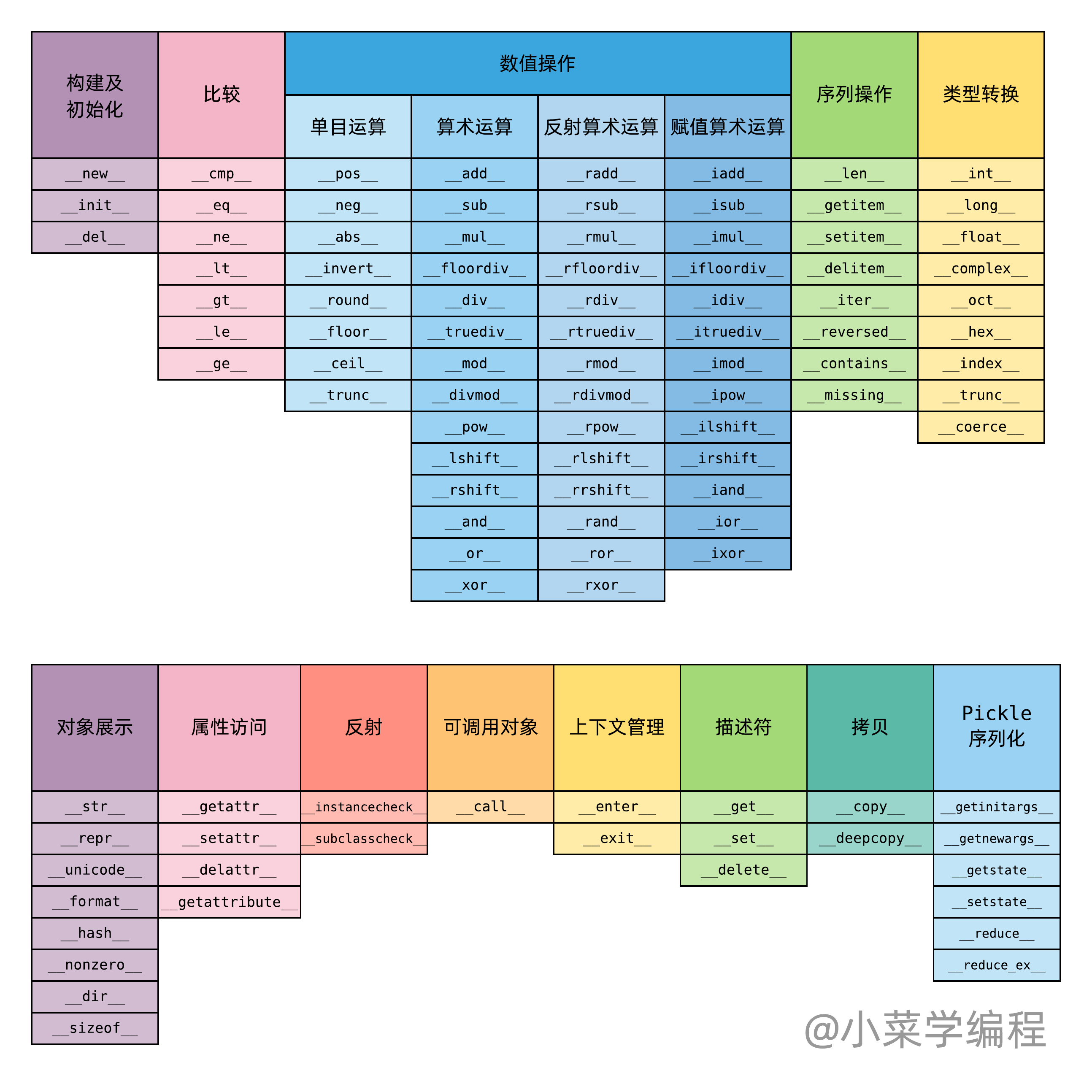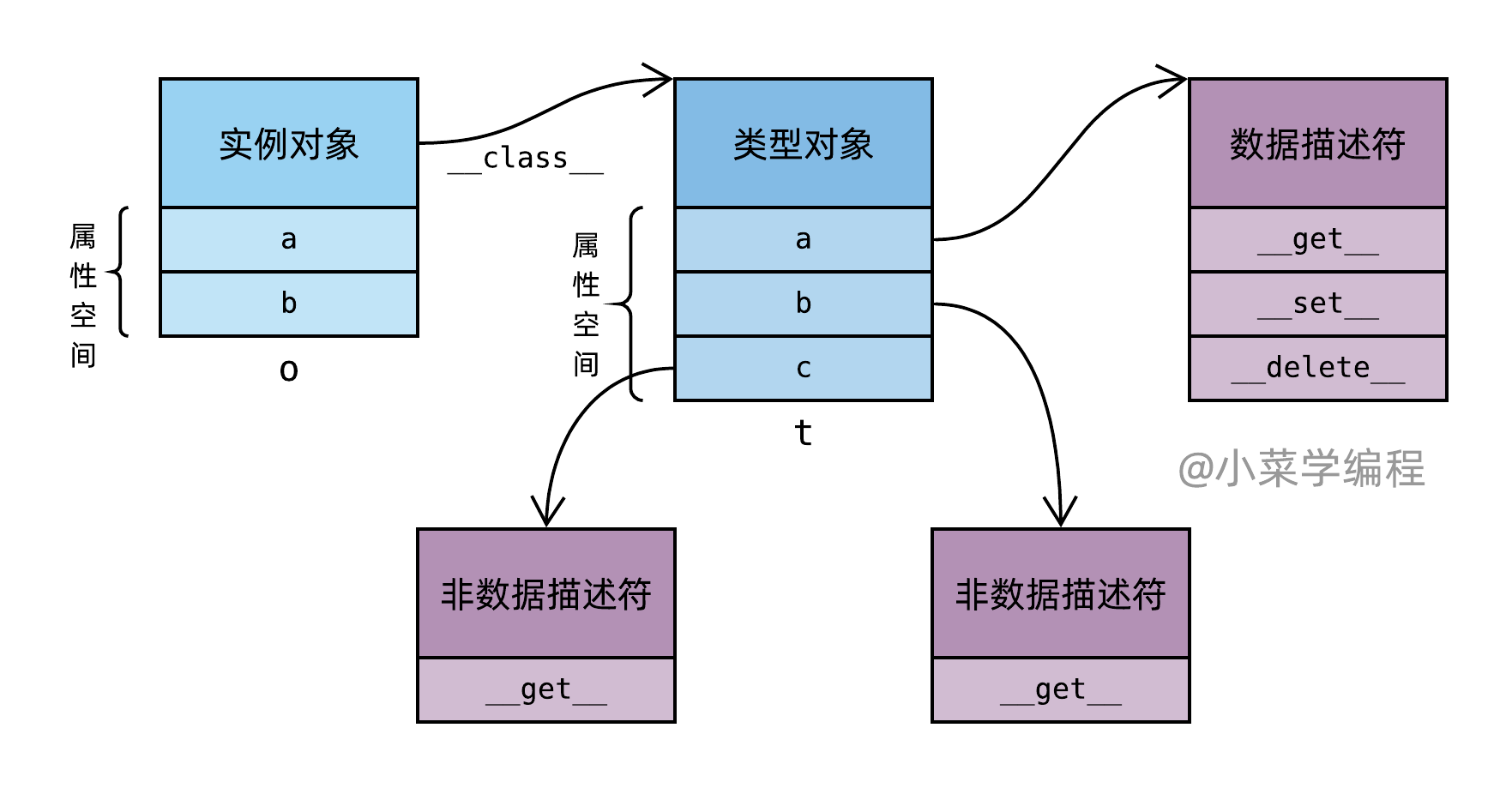# 高阶编程：魔术方法应用实战## 运算符重载

 1 2 3 4 5 6 7 8  >>> 'hello' + ' ' + 'world' 'hello world' >>> 'abc' * 10 'abcabcabcabcabcabcabcabcabcabc' >>> 'hello world' / ' ' Traceback (most recent call last): File "", line 1, in TypeError: unsupported operand type(s) for /: 'str' and 'str' 

 1 2 3 4  class SmartString(str): def __truediv__(self, other): return self.split(other) 

Python 内部，除法操作 / 由 truediv 魔术方法处理。请注意，在老版本 Python 中，除法操作符由 div 魔术方法处理，名字略有差异。

 1 2 3  >>> s = SmartString('hello world') >>> s / ' ' ['hello', 'world'] 

## 数值型运算

  1 2 3 4 5 6 7 8 9 10 11 12 13 14  class Vector: def __init__(self, x, y): self.x = x self.y = y def __add__(self, other): return Vector(x=self.x+other.x, y=self.y+other.y) def __sub__(self, other): return Vector(x=self.x-other.x, y=self.y-other.y) def __mul__(self, scale): return Vector(x=self.x*scale, y=self.y*scale) def __truediv__(self, scale): return Vector(x=self.x/scale, y=self.y/scale) def __repr__(self): return 'Vector(x={}, y={})'.format(self.x, self.y) 
• sub 魔术方法，实现向量减法；
• mul 魔术方法，实现向量数乘；
• truediv 魔术方法，实现向量数除；
• repr 魔术方法，实现向量表示；

 1 2 3 4 5 6 7 8  >>> v1 = Vector(1, 2) >>> v2 = Vector(3, 4) >>> v1 + v2 Vector(x=4, y=6) >>> v1 * 3 Vector(x=3, y=6) >>> v1 / 2 Vector(x=0.5, y=1.0) 

 1 2 3 4 5  >>> v1 = Vector(1, 2) >>> v2 = Vector(3, 4) >>> v3 = Vector(10, 2) >>> sum((v1, v2, v3), Vector(0, 0)) Vector(x=14, y=8) 

## 属性描述符

• 非数据描述符 ：只实现了 get 方法；
• 数据描述符 ：至少实现了 set 或者 delete 方法；

1. 数据描述符：如果类型对象(含父类)定义了同名数据描述符属性，属性操作将被其接管；
2. 对象属性：除了①，属性操作默认在属性空间中完成；
3. 非数据描述符：属性访问时，如果①②均不成功，而类型对象(含父类)定义了同名非数据描述符，属性访问将被其接管；• 对于属性 a ，由于类型对象 t 属性空间定义了数据描述符，将屏蔽实例对象 o 属性空间中的定义；
• 对于属性 b ，由于类型对象 t 属性空间定义的只是非数据描述符，仍以实例对象 o 属性空间定义的为准；
• 对于属性 c ，由于实例对象 o 属性空间未定义，属性访问将以类型对象 t 属性空间定义的非数据描述符为准；
• 对于属性 c ，由于类型对象 t 属性空间定义的只是非数据描述符，属性设置、删除仍以实例对象 o 属性空间为准；

【Python源码剖析】系列文章首发于公众号【小菜学编程】，敬请关注：【Python源码剖析】系列文章首发于公众号【小菜学编程】，敬请关注：